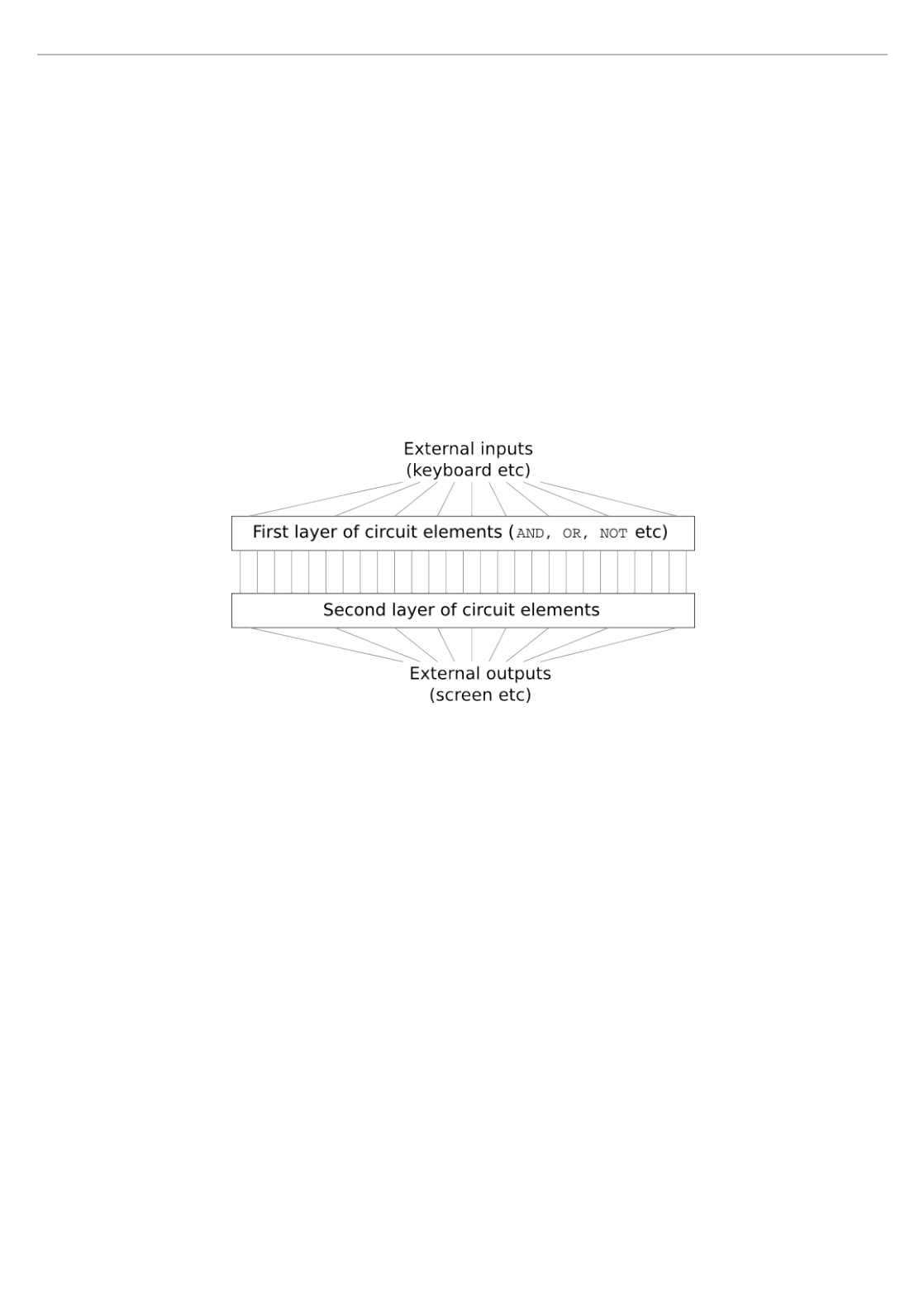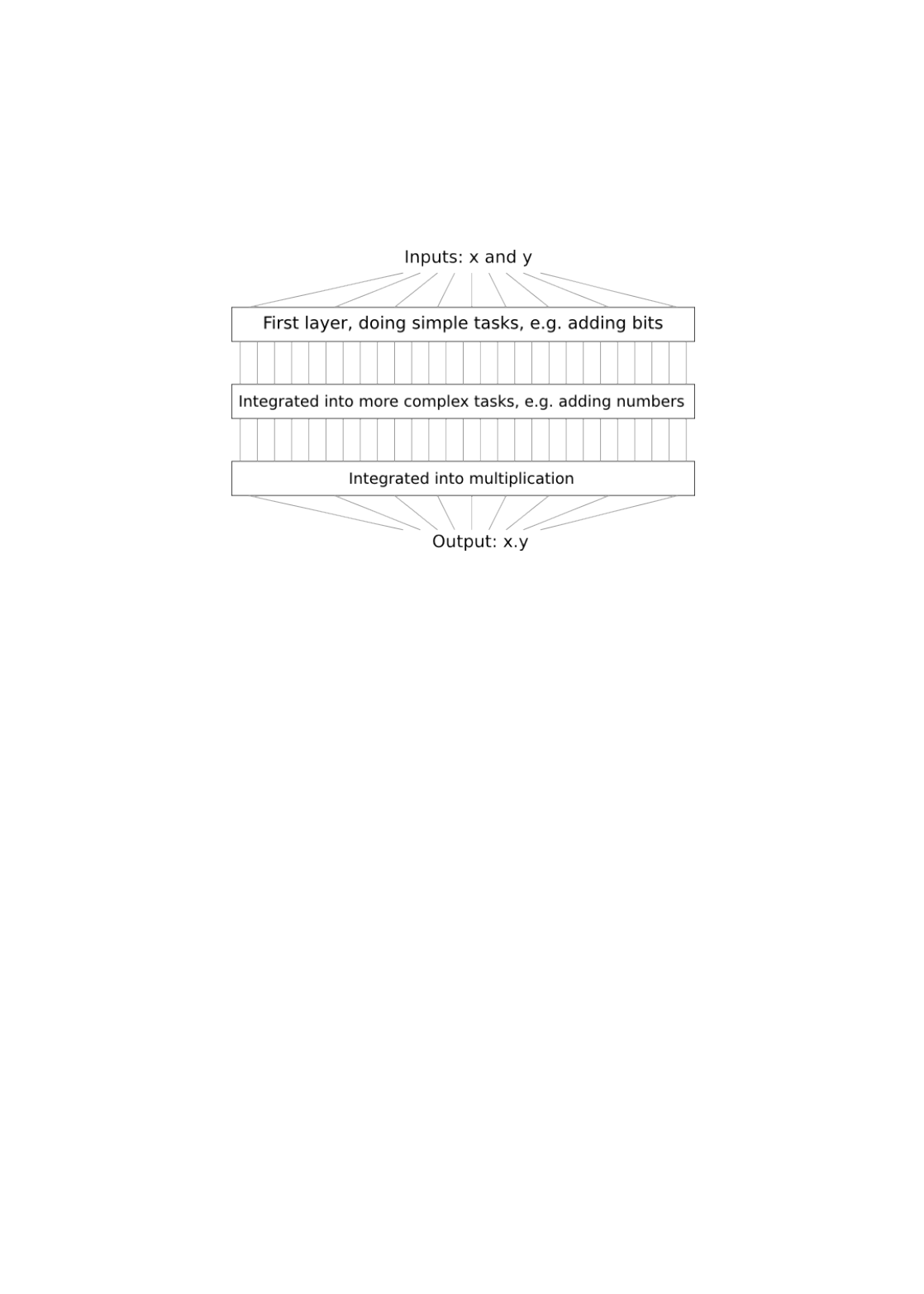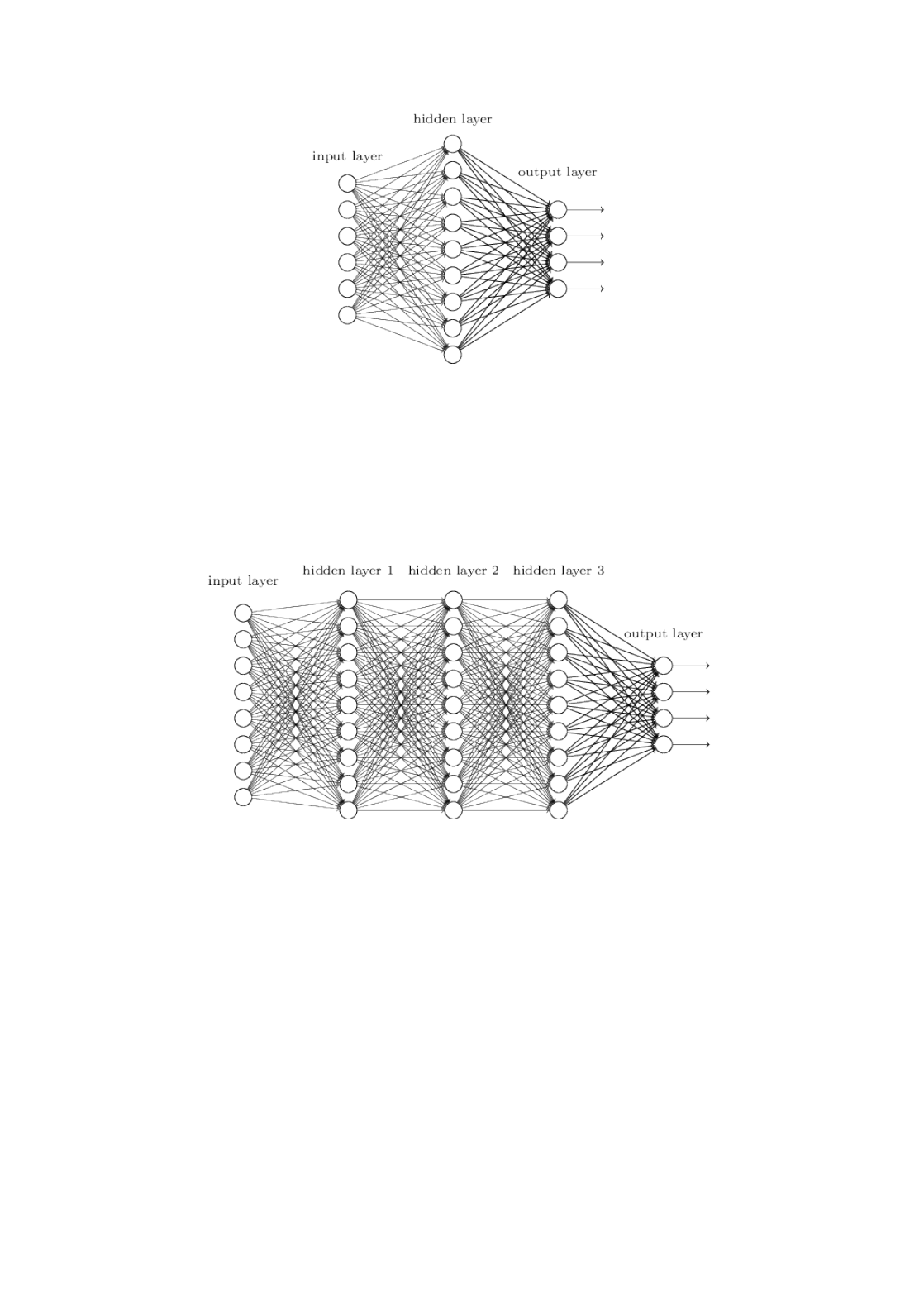Imagine you're an engineer who has been asked to design a
computer from scratch. One day you're working away in your
office, designing logical circuits, setting out AND gates, OR
gates, and so on, when your boss walks in with bad news. The
customer has just added a surprising design requirement: the
circuit for the entire computer must be just two layers deep:
You're dumbfounded, and tell your boss: "The customer is
crazy!"
Your boss replies: "I think they're crazy, too. But what the
customer wants, they get."
In fact, there's a limited sense in which the customer isn't
crazy. Suppose you're allowed to use a special logical gate
which lets you AND together as many inputs as you want. And
you're also allowed a many-input NAND gate, that is, a gate
which can AND multiple inputs and then negate the output.
With these special gates it turns out to be possible to compute
any function at all using a circuit that's just two layers deep.
But just because something is possible doesn't make it a good
idea. In practice, when solving circuit design problems (or most
any kind of algorithmic problem), we usually start by figuring
out how to solve sub-problems, and then gradually integrate
the solutions. In other words, we build up to a solution through
CHAPTER 5
Why are deep neural networks hard to train?multiple layers of abstraction.
For instance, suppose we're designing a logical circuit to
multiply two numbers. Chances are we want to build it up out
of sub-circuits doing operations like adding two numbers. The
sub-circuits for adding two numbers will, in turn, be built up
out of sub-sub-circuits for adding two bits. Very roughly
speaking our circuit will look like:
That is, our final circuit contains at least three layers of circuit
elements. In fact, it'll probably contain more than three layers,
as we break the sub-tasks down into smaller units than I've
described. But you get the general idea.
So deep circuits make the process of design easier. But they're
not just helpful for design. There are, in fact, mathematical
proofs showing that for some functions very shallow circuits
require exponentially more circuit elements to compute than
do deep circuits. For instance, a famous series of papers in the
early 1980s*
*The history is somewhat complex, so I won't give detailed references. See
Johan Håstad's 2012 paper On the correlation of parity and small-depth
circuits for an account of the early history and references.
showed that computing the parity of a set of bits requires
exponentially many gates, if done with a shallow circuit. On the
other hand, if you use deeper circuits it's easy to compute the
parity using a small circuit: you just compute the parity of pairs
of bits, then use those results to compute the parity of pairs of
pairs of bits, and so on, building up quickly to the overall
parity. Deep circuits thus can be intrinsically much more
powerful than shallow circuits.
Up to now, this book has approached neural networks like thecrazy customer. Almost all the networks we've worked with
have just a single hidden layer of neurons (plus the input and
output layers):
These simple networks have been remarkably useful: in earlier
chapters we used networks like this to classify handwritten
digits with better than 98 percent accuracy! Nonetheless,
intuitively we'd expect networks with many more hidden layers
to be more powerful:
Such networks could use the intermediate layers to build up
multiple layers of abstraction, just as we do in Boolean circuits.
For instance, if we're doing visual pattern recognition, then the
neurons in the first layer might learn to recognize edges, the
neurons in the second layer could learn to recognize more
complex shapes, say triangle or rectangles, built up from edges.
The third layer would then recognize still more complex
shapes. And so on. These multiple layers of abstraction seem
likely to give deep networks a compelling advantage in learning
to solve complex pattern recognition problems. Moreover, just
as in the case of circuits, there are theoretical results suggesting
that deep networks are intrinsically more powerful than
shallow networks*
*For certain problems and network architectures this is proved in On thenumber of response regions of deep feed forward networks with piece-wise
linear activations, by Razvan Pascanu, Guido Montúfar, and Yoshua Bengio
architectures for AI, by Yoshua Bengio (2009).
.
How can we train such deep networks? In this chapter, we'll try
training deep networks using our workhorse learning
algorithm - stochastic gradient descent by backpropagation.
But we'll run into trouble, with our deep networks not
performing much (if at all) better than shallow networks.
That failure seems surprising in the light of the discussion
above. Rather than give up on deep networks, we'll dig down
and try to understand what's making our deep networks hard
to train. When we look closely, we'll discover that the different
layers in our deep network are learning at vastly different
speeds. In particular, when later layers in the network are
learning well, early layers often get stuck during training,
learning almost nothing at all. This stuckness isn't simply due
to bad luck. Rather, we'll discover there are fundamental
reasons the learning slowdown occurs, connected to our use of
As we delve into the problem more deeply, we'll learn that the
opposite phenomenon can also occur: the early layers may be
learning well, but later layers can become stuck. In fact, we'll
find that there's an intrinsic instability associated to learning
by gradient descent in deep, many-layer neural networks. This
instability tends to result in either the early or the later layers
getting stuck during training.
This all sounds like bad news. But by delving into these
difficulties, we can begin to gain insight into what's required to
train deep networks effectively. And so these investigations are
good preparation for the next chapter, where we'll use deep
learning to attack image recognition problems.
So, what goes wrong when we try to train a deep network?
To answer that question, let's first revisit the case of a network
with just a single hidden layer. As per usual, we'll use the
MNIST digit classification problem as our playground forlearning and experimentation*
*I introduced the MNIST problem and data here and here.
.
If you wish, you can follow along by training networks on your
computer. It is also, of course, fine to just read along. If you do
wish to follow live, then you'll need Python 2.7, Numpy, and a
copy of the code, which you can get by cloning the relevant
repository from the command line:
git clone https://github.com/mnielsen/neural-networks-and-deep-learning.git
If you don't use git then you can download the data and code
here. You'll need to change into the src subdirectory.
Then, from a Python shell we load the MNIST data:
>>> training_data, validation_data, test_data = \
We set up our network:
>>> import network2
>>> net = network2.Network([784, 30, 10])
This network has 784 neurons in the input layer,
corresponding to the pixels in the input image.
We use 30 hidden neurons, as well as 10 output neurons,
corresponding to the 10 possible classifications for the MNIST
digits ('0', '1', '2', , '9').
Let's try training our network for 30 complete epochs, using
mini-batches of 10 training examples at a time, a learning rate
, and regularization parameter . As we train we'll
monitor the classification accuracy on the validation_data*
*Note that the networks is likely to take some minutes to train, depending on
the speed of your machine. So if you're running the code you may wish to
continue reading and return later, not wait for the code to finish executing.
:
>>> net.SGD(training_data, 30, 10, 0.1, lmbda=5.0,
... evaluation_data=validation_data, monitor_evaluation_accuracy=True)
We get a classification accuracy of 96.48 percent (or
thereabouts - it'll vary a bit from run to run), comparable to our
earlier results with a similar configuration.
Now, let's add another hidden layer, also with 30 neurons in it,
28 × 28 = 784
η = 0.1
λ = 5.0and try training with the same hyper-parameters:
>>> net = network2.Network([784, 30, 30, 10])
>>> net.SGD(training_data, 30, 10, 0.1, lmbda=5.0,
... evaluation_data=validation_data, monitor_evaluation_accuracy=True)
This gives an improved classification accuracy, 96.90 percent.
That's encouraging: a little more depth is helping. Let's add
another 30-neuron hidden layer:
>>> net = network2.Network([784, 30, 30, 30, 10])
>>> net.SGD(training_data, 30, 10, 0.1, lmbda=5.0,
... evaluation_data=validation_data, monitor_evaluation_accuracy=True)
That doesn't help at all. In fact, the result drops back down to
96.57 percent, close to our original shallow network. And
suppose we insert one further hidden layer:
>>> net = network2.Network([784, 30, 30, 30, 30, 10])
>>> net.SGD(training_data, 30, 10, 0.1, lmbda=5.0,
... evaluation_data=validation_data, monitor_evaluation_accuracy=True)
The classification accuracy drops again, to 96.53 percent.
That's probably not a statistically significant drop, but it's not
encouraging, either.
This behaviour seems strange. Intuitively, extra hidden layers
classification functions, and thus do a better job classifying.
Certainly, things shouldn't get worse, since the extra layers can,
in the worst case, simply do nothing*
*See this later problem to understand how to build a hidden layer that does
nothing.
. But that's not what's going on.
So what is going on? Let's assume that the extra hidden layers
really could help in principle, and the problem is that our
learning algorithm isn't finding the right weights and biases.
We'd like to figure out what's going wrong in our learning
algorithm, and how to do better.
To get some insight into what's going wrong, let's visualize how
the network learns. Below, I've plotted part of a
network, i.e., a network with two hidden layers, each
containing hidden neurons. Each neuron in the diagram has
a little bar on it, representing how quickly that neuron is
changing as the network learns. A big bar means the neuron's
weights and bias are changing rapidly, while a small bar means
[784, 30, 30, 10]
30